# Applied fantasy math – Vampire populations

Some time ago, I became aware of a nice paper – The Transylvanian problem of renewable resources by R.Hartl and A. Mehlmann. You can find an ungated copy here. The paper itself is quite technical and I’m not familiar enough with optimal control theory to fully explore it here. The idea of the paper however is to consider a vampire population feeding on humans and find optimal feeding strategies for different discountings of consumption.

H&M assume the following model for the vampire-human population: Vampires consume human blood and each such human becomes another vampire. Vampires are also lost to sunlight, vampire hunters, crosses etc. Thus, the number of vampires changes according to$$\dot{v} = -a v(t) + c v(t),$$where a and c model amount of vampires lost and amount of feeding respectively and $\dot{v}$ denotes a derivative with respect to time. For example, if a=0.1, this means that for each time unit, 10% of vampires perish. Naturally a and c can be arbitrary functions. For humans, we have a similar equation $$\dot{h} = nh(t) -cv(t),$$ where n is the humans growth rate and c is the familiar vampire feeding rate. Here new humans are born and some of them are lost to vampire attacks. Note that while this model does not have other means of perishing explicit, they are included in the formulation of n. It should also be noted that here, each feeding results in a new vampire and a dead human.

There are two complications. First, vampires very likely prefer consumption now to consumption tomorrow. This is very reasonable, since future consumption is much more uncertain than consumption now. Secondly, the can have rising or lowering marginal utility of blood. In the case of rising marginal utility, vampires get more utility from feeding twice than two feedings of one and in the case of lowering marginal utility, the situation is inverse. The conclusion they reach is that rising and constant marginal utility vampires optimally feed out all humans. The same happens for lowering marginal utility vampires unless the discount rate is sufficiently low i.e. that the vampires appreciate future consumption enough – conservationist vampires, if you may.

However, this was mostly introduction to suck you in… Ahem. To get you interested. As you might have noted, the vampire-human model is quite simple and it’s very easy to play with the parameters. If you want to see for yourself, I’ve attached a couple of very simple python3 scripts at the end of the post.

First, consider constant feeding rates. Below, I’ve plotted a few different possibilities.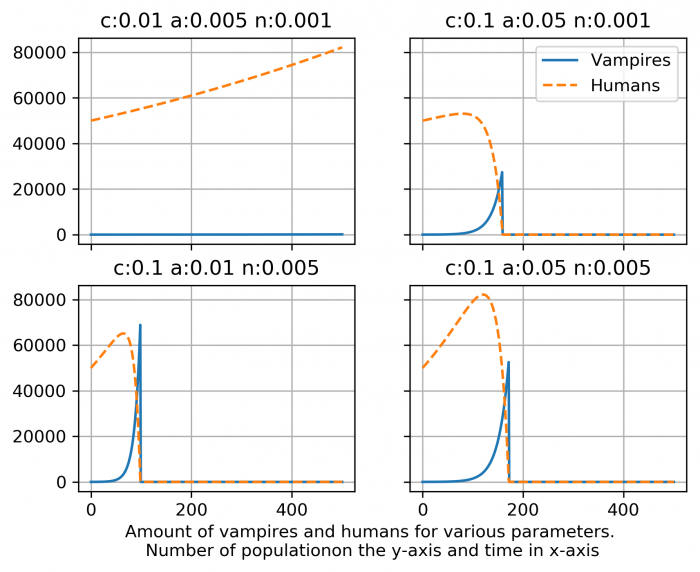As you see, it’s very easy to feed out all humans. In this kind of model, it makes sense for the vampires to self regulate very hard, given that they have potential for very long lives. Also note that the line in the upper left case is not zero, but the vampire population is kept small. The feeding rate is very small and not much larger than the ‘expiration’ rate.

The paper then continues to to prove a few properties with this model, but we might be interested in tinkering with this model. For example, instead of constants, we can also put in arbitrary functions of t,v and h:$$\dot{v} = -a(v,h,t)\cdot v(t) + c(v,h,t)\cdot v(t),$$$$\dot{h} = n(v,h,t)\cdot h(t) -c(v,h,t)\cdot v(t),$$However, in such a case, the differential equations might not be easily solvable.  This can be worked around by considering a system with discrete time steps – the state of the system at the time step t-1 determines the system’s state at time t. Then the evolution of the system – i.e. in this case, the number of vampires at time t would be following: $$v(t)= v(t-1) -a(t-1)\cdot v(t-1) + c(t-1)\cdot v(t-1),$$and similarly for humans. A simple modification we could make is that if the vampire population is more than 10% of human population, vampires reduce their feeding to 0.01 at each time step until human population catches up and if there are enough humans to go around, the vampires increase their feeding (and thus also new vampires) but 10% at each time step. The resulting vampire population would look like this: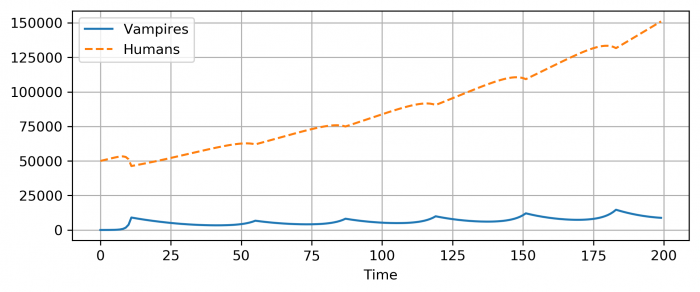With this formulation it is extremely easy to consider different variations, but perhaps most information is already present in the simple model.

By now, you might be wondering, what is the point of all this, besides a mathematical exercise and a few solutions for renewable resources. Well, first, there is an appropriate Richard Feynman quote, but the second reason is, it might inform you on what kind of vampire societies might exist in your games.

Obviously the model  is only a model and it does not have very many moving parts, but I still think it’s interesting to explore even such simple toy models. For example, we get yet another example where exponential growth can blow up. For story/worldbuilding purposes, this gives a bit more weight to various secrecy schemes vampires are usually associated with. Now, this is all fine and good, but to be honest, I just saw a funny paper and decided to play with the model a little. 🙂

I will also say that To the readers concerned that the authors were irresponsibly aiding forces of darkness, I would like to mention a follow up paper by Dennis Snower – Macroeconomic policy and optimal destruction of vampires. I quote the following comment on the original article, but do read the whole thing.

“One wonders what conceivable interest the authors could have had in helping vampires solve their intertemporal consumption problem. The implicit assumption of the Invisible Hand (or Fang)-whereby vampires, in pursuing their own interests, pursue those of human beings as well-is of questionable validity. “

For those interested, I attached the scripts I used for this blog below. Although, if you can get them to run (python3 + a couple of standard packages), I suspect you could easily write them yourself

 Source code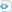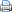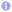import matplotlib.pyplot as plt
import numpy as np

#First let's define some parameters
#Starting population and maximum time
h0 = 50000
v0 = 10
tmax = 500

#Model parameters
n = 0.01                #new humans, i.e. h(t+1) = n*h(t)
c = 0.5               #Feeding rate
a = 0.05                #vampire loss rate

def vampires(t):
vamp = v0 * np.exp((c -a) * t)
#A limit so that we can't have negative number of vampires
return np.max([vamp,0])

def humans(t):
hum = (np.exp(n*t)*((a*h0 + (n - c)*h0) + c * (np.exp((c - a - n)*t) - 1)* v0)) / (a - c + n)
#No negative number of humans
return np.max([hum, 0])

#Generate populations
t = np.linspace(0, tmax, 500)
v = [vampires(time) for time in t]
h = [humans(time) for time in t]

# Just a sanity test to check that the functions give
# correct outputs for t=0
print("h0: " + str(humans(0)))
print("v0: " + str(vampires(0)))
print("h(tmax): " + str(humans(tmax)))
print("v(tmax): " + str(vampires(tmax)))

plt.figure()
plt.plot(t, v, '-', label="Vampires")
plt.plot(t, h, '--', label="Humans")
plt.grid()
plt.title("Parameters \n" +
"a:" + str(a) + " c:" + str(c) + " n:" + str(n) + "\n" +
"h0:" + str(h0) + " v0:" + str(v0))
plt.xlabel("Time")
plt.legend(numpoints = 1)
#plt.savefig("vampires_humans.png", dpi=300, bbox_inches='tight')
plt.show()

And then the discrete version.

 Source codeimport matplotlib.pyplot as plt
import numpy as np

#Starting populations
h0 = 50000
v0 = 10
#Maximum time, from 0 to tmax steps
tmax = 100

n0 = 0.005               #new humans, i.e. h(t+1) = n*h(t)
c0 = 0.1               #Feeding rate
a0 = 0.01            #vampire loss rate

#Define functions to get population at time t
def vampires(vprev, c, a):
vamp = vprev +  c * vprev - a * vprev
#A limit such that amount of vampires can't be negative
return np.max([vamp,0])

def humans(vprev, hprev, c, n):
hum = hprev + n * hprev - c * vprev
#Same for humans
return np.max([hum,0])

#generate populations:
v = [v0]
h = [h0]
t = 
i = 1
# _0 variables to keep track of the original values,
#if we introduce some changes in them and want
#to restore the original value.
c = c0
a = a0
n = n0

#Generate the populations
while(i < tmax):
v.append(vampires(v[i-1], c, a))
h.append(humans(v[i-1], h[i-1], c, n))
t.append(i)
i += 1

#Plot the results
fig = plt.figure()
plt.plot(t, v, '-', label="Vampires")
plt.plot(t, h, '--', label="Humans")
plt.grid()
plt.xlabel("Time")
plt.legend(numpoints = 1)
fig.set_size_inches(8,3)
#plt.savefig("vampires_humans_discrete_hunters.png", dpi=300, bbox_inches='tight')
plt.show()